## research papersBIOLOGICALCRYSTALLOGRAPHY
ISSN: 1399-0047
Volume 56| Part 10| October 2000| Pages 1270-1277

## Resolution measurement in structures derived from single particles

aHoward Hughes Medical Institute, W. M. Keck Institute for Cellular Visualization, Rosenstiel Basic Medical Sciences Research Center, Department of Biochemistry, Brandeis University, 415 South Street, Waltham, MA 02454-9110, USA
*Correspondence e-mail: niko@brandeis.edu

(Received 4 February 2000; accepted 16 July 2000)

Analytical expressions are derived and computer simulations are presented to assess the accuracy of procedures commonly used to estimate the resolution of three-dimensional (3D) structures derived from images of single protein molecules or complexes. It is shown that in the case of a low signal-to-noise ratio in the images, the Fourier ring correlation between two structures, each calculated using one half of the data, significantly overestimates the resolution when the two half data sets were aligned against the same reference structure. The overestimate arises because of a correlation between the noise components present in the images. The correlation is introduced by the alignment and becomes more serious as the signal-to-noise ratio is reduced. A reliable resolution measure is only obtained when the two half data sets are aligned against two independent reference structures. It is further shown that the noise correlation also significantly affects the spectral signal-to-noise ratio and the Q factor, making them unreliable measures of signal present in a 3D structure and in the original images, respectively. It is concluded that the alignment of images is always accompanied by a correlation of the noise and that this correlation is indistinguishable from a correlation arising from a signal.

### 1. Introduction

Electron microscopy of biological macromolecules has become a powerful technique for determining the three-dimensional (3D) structure of proteins and protein complexes. The development of crystallographic methods and their application to two-dimensional (2D) crystals produce 3D density maps at 3–4 Å resolution which can be interpreted by atomic models (Henderson et al., 1990; Kühlbrandt et al., 1994; Nogales et al., 1998). At the same time, electron microscopy of isolated protein molecules and complexes (single particles) continues to progress to higher resolution and has yielded 3D maps at 7 Å resolution in the case of highly symmetrical viruses (Böttcher et al., 1997; Conway et al., 1997) and 11.5 Å resolution for the asymmetrical ribosome (Gabashvili et al., 2000).

The physical limits of structure determination using single particles have been discussed (Henderson, 1995) and it appears possible to obtain a density map at 3 Å resolution if the particle mass is sufficiently large (300–4000 kDa, depending on the attainable contrast in the electron microscope) and given a sufficiently large number of images to average over (104–106 images). It is essential to use a reliable resolution measure to judge the progress in resolution made with larger amounts of data and new techniques. Commonly, a correlation coefficient is used to measure the resolution of a 3D map calculated from single-particle images. For this purpose, the set of images is divided into two subsets, each containing one half of the images of the complete set. The distribution of images between the two sets should be random, but in practice they are usually divided into odd and even-numbered particles. Two 3D maps are calculated from the subsets and their Fourier transformations, F1 and F2, are computed. The resolution of the two maps is then estimated by the Fourier shell correlation (FSC; Harauz & van Heel, 1986),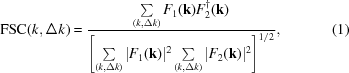which is evaluated for each resolution shell (k,Δk). Here, † denotes the complex conjugate. For pure noise the expectation value for the FSC is 1/[N(k,Δk)]1/2, where N(k,Δk) is the number of terms in the shell. The resolution cutoff is then often taken at the point where FSC < 2/[N(k,Δk)]1/2 (Frank, 1996). It is important to note that the assumption made with the above correlation analysis is that the two subsets are independent of each other. However, this is usually not true for reasons outlined in the following.

When working with images of single particles, the main task is to determine the orientation (three angles) and position (two coordinates) of each particle as accurately as possible. These parameters can be determined, for example, using a 3D map of the particle as a reference. Projections from such a 3D reference map can be generated in directions uniformly sampling all possible orientations and the closest match for each particle image can then be found (Penczek et al., 1994). Such an approach represents a parameter search and is limited in accuracy to half the step width used. A problem arises at the beginning, when no 3D map of the particle is available. Usually, a first map is generated using multivariate statistical analysis and classification of the image set, which results in class averages representing common views of the particle. The relative orientation of these views can then be determined using, for example, the angular reconstitution method (van Heel, 1987).

Once the orientation and position of each particle is approximately determined and a first 3D map has been calculated, the parameters are refined. Refinement differs from a parameter search in that the accuracy of the determined parameters is not limited by a step width. Instead, a function is maximized or minimized depending on the function used. For example, one could maximize a cross-correlation coefficient between an image and a projection of the reference map by varying the angles determining the direction of projection. With the new parameters, a better 3D map can be calculated and used in the next refinement cycle. The iteration is terminated when the parameters stop changing significantly between cycles.

Since in this iterative procedure the parameters for each particle are refined against the same reference, the two subsets of images used in the correlation analysis to obtain a resolution estimate are not independent. As will be shown, this can lead to completely false resolution estimates.

### 2. False correlation of sums of images aligned to a common reference

Given is a set of M images of particles, each with N = n × n pixels, and each image is normalized to have an average of zero and a variance of one. For simplicity, we assume that (i) we are only interested in a 2D projection map of our particle, (ii) that the images all show the same view of our particle and (iii) that the particles are rotationally aligned with each other and only need to be aligned in their two positional coordinates. We would like to monitor the progress of our parameter refinement by means of a correlation coefficient CC with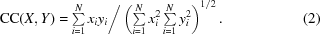X and Y are two images with pixel values xi and yi, respectively. In (2) we used the fact that the pixel averages of xi and yi are zero. Again, for the sake of simplicity, we calculate a correlation coefficient in real space between the averages of two subsets of images. This correlation coefficient equals the spectral average of the Fourier ring correlation (FRC; Saxton & Baumeister, 1982; van Heel et al., 1982), which is the two-dimensional equivalent of the FSC.

What happens when images contain pure noise? We know that if we calculate the correlation coefficient between two of the noise images, the expectation value of the correlation coefficient is zero. However, if we align the two images to each other to maximize the correlation coefficient, the expectation value of the maximum will be greater than zero. The variance of the correlation coefficient for pure noise is σ2 = 1/N and for large N its distribution is approximately normal. If we allow shifts in the two positional coordinates to be applied to either of the two images, we can calculate N′ = N different correlation coefficients. For large N′, the asymptotic expectation value of the maximum of N′ normally distributed correlation coefficients with a variance 1/N is (Grigorieff & Grigorieff, 1999)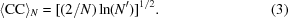(3) gives the correlation coefficient if we align two pure noise images, each having N = n × n pixels, in their two positional coordinates. For large N, the expectation value approaches zero, as expected.

Now we expand the equations for the case of M images. We assume we have all M images aligned to each other and we indicate an aligned image or image coordinate by *. We note that as a consequence of the alignment the covariance λ of pixel values is the same for any two images X* and Y*: λ = cov(X*, Y*) > 0. We select an image Xn* and calculate the correlation coefficient between the sum of the remaining M − 1 images and image Xn*. The expectation value of the correlation coefficient will be the same as that between two single aligned noise images, since the sum of M − 1 images is again a noise image. We write for the correlation coefficient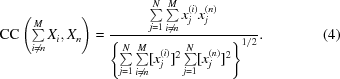To calculate the expectation value of the correlation coefficient we use the property of the expectation value of the quotient of two random variables x and y, which can be approximated as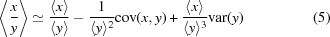(Mood et al., 1974). We observe that for large N the variance of the denominator in (4) and its covariance with the numerator are small compared with the mean of the denominator. Thus,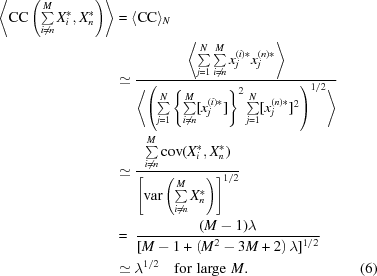In (6) we have observed again that all images are normalized with zero mean and a variance of one. Also, to calculate the expectation value of the denominator we again used the fact that the variance of the double sum under the square root is small compared with its mean.

When we split the data set into two, each containing M/2 of the aligned images, the expectation value 〈CC〉N,M of the correlation coefficient between the two sums is given byHere and in (6) we used the relations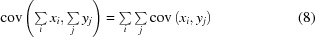and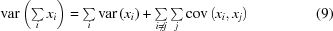(Mood et al., 1974). We note that as the number of images M approaches infinity we expect a correlation coefficient of 1. Therefore, if all images are aligned against a common reference, the correlation coefficient is not a reliable measure for the signal present in the average. For example, if we have 1000 images of size N = 64 × 64 pixels we will find a correlation of 0.67; for 5000 images we find a correlation of 0.91. If we allow rotational alignment of the images in addition to the alignment in their two positional coordinates we expect the situation to become worse; the number of independent correlation coefficients N′ in (3) will be larger and hence 〈CC〉N will increase, leading in turn to an increase in 〈CC〉N,M.

Similar arguments can be made for images that are used to calculate a 3D reconstruction of a particle. The correlation coefficient for testing the resolution will be calculated between two 3D maps, each containing a number of voxels which is larger than the number of pixels in the two-dimensional case. However, the number of degrees of freedom in the alignment is also larger and includes the two positional coordinates as well as the three angles.

### 3. Other measures of resolution

#### 3.1. Spectral signal-to-noise ratio

The spectral signal-to-noise ratio (SSNR) has been suggested as another resolution measure (Unser et al., 1987, 1989). It is defined as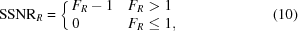with the spectral variance ratio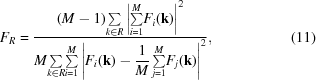where Fi is the Fourier transform of image Xi and R is the region in Fourier space for which the SSNR is evaluated. The expectation value of FR is one for images containing pure uncorrelated noise (Unser et al., 1987). We note that for images containing pure noise the probability distribution is the same for all Fourier coefficients. The expectation value of each Fourier coefficient is zero, the variance of the Fourier coefficients is related to the variance in the image by ­var(Fi) = N­var(Xi) and the covariance of coefficients of two Fourier transforms is cov(Fi,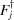) = Ncov(Xi, Xj). When calculating the expectation value of FR for a series of M aligned images, we observe that for large M the numerator and denominator can be treated independently as before,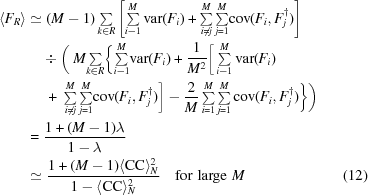and hence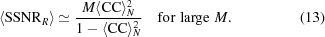(13) gives an average for the SSNR over the entire spectrum. For large M, we expect the SSNR to be significantly larger than one. Hence, the SSNR shows the same effect of spuriously high values. For example, if we have 1000 images of size N = 64 × 64 pixels, we will find a SSNR of 4.1; for 5000 images, we find a SSNR of 20.4. The relation between the correlation coefficient and the SSNR is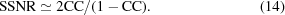(14) is identical to the relation given by Frank & Al-Ali (1975) except for a factor of 2, which accounts for the fact that the SSNR in (14) describes the signal-to-noise ratio of the final average of all images rather than that of the averages of the two half sets that are being compared by the correlation coefficient.

#### 3.2. Q factor

Another measure of resolution is given by the Q factor (van Heel & Hollenberg, 1980; Kessel et al., 1985), defined as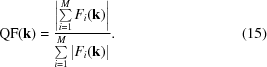k is a particular location (pixel) in the Fourier transform for which the Q factor is calculated. The Q factor is zero for pure uncorrelated noise and one for a noise-free signal. As before, the expectation value of QF can be approximated by calculating the expectation values for the numerator and denominator separately. We note that the denominator obeys Wilson statistics (Wilson, 1949) and write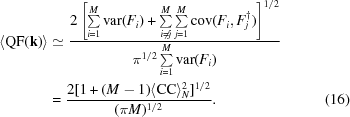(16) gives an average for the Q factor over the entire spectrum. For large M, the Q factor approaches the value of (2/π1/2)〈CC〉N. This means that the Q factor remains small even for aligned noise images. For example, for images of size N = 64 × 64 pixels we have a Q factor of 0.072 in the limit of an infinite number of images. (16) shows that the Q factor is essentially independent of the number of images in the data set and only depends on their mutual correlation. Thus, it measured the signal present in the original images and not in the final average. Since we have a non-zero correlation of pure noise images after their alignment, the Q factor is also not zero and suffers from the same false indication of signal as the quantities discussed before.

### 4. Simulations on the computer

#### 4.1. Pure noise

To validate the expressions for the FRC, SSNR and Q-­factor simulations were carried out on a computer using the image-processing package SPIDER (Frank et al., 1996). Three data sets consisting of 1000, 5000 and 10 000 images containing 64 × 64 pixels of normally distributed noise (unit variance and zero average) were generated. 30 cycles of translational alignment were executed for each data set. One cycle consisted of calculation of the average of all images using the alignment parameters from the previous cycle and subsequent alignment of all images to the new average using a cross-correlation function. For the first cycle, the original noise images were used to calculate the first average. Fig. 1shows plots of the FRC, SSNR and Q-factor values in resolution zones for all data sets; Table 1gives values averaged over the entire spectrum. The calculated averages agree well with those found in the simulations. Fig. 1shows that the values for the FRC, SSNR and Q factor are approximately constant across the spectrum and that the noise in the curves increases for smaller data sets.

 Table 1Comparison of simulations and calculations
 Spectral average values for the FRC, SSNR and Q factor for three different data sets and calculated values using the equations derived in the text.
FRC SSNR Q factor
Data set Simulation Simulation Simulation
N = 1000 0.67 0.67 3.89 4.06 0.070 0.080
N = 5000 0.89 0.91 18.4 20.4 0.063 0.074
N = 10000 0.95 0.95 35.6 40.8 0.061 0.073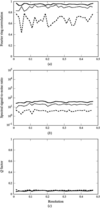Figure 1 (a) FRC, (b) SSNR and (c) Q factor in resolution zones for aligned data sets with images containing only normally distributed noise. The data sets contained M = 1000 (dashed lines), 5000 (dotted lines) and 10 000 images (unbroken lines). The noise in the plots is highest for the smallest data set. All plots show approximately constant values across the spectrum. The resolution is given in units of pixel−1.

#### 4.2. The presence of signal

When signal is present in the images the expectation values of the resolution measures change. This is the result of a reduced degree of freedom in the alignment: the signal will place constraints on the alignment of individual images by favoring defined positions which are independent of the noise. If the magnitude of the signal is not constant across the Fourier spectrum, the statistics of the expectation values will also vary across the spectrum. The final alignment will depend on both the signal-to-noise ratio and the spectral distribution of the signal. A test pattern was generated (Fig. 2a) and normalized (unit variance and zero mean). The average amplitude in resolution zones of the Fourier spectrum of the test pattern is shown in Fig. 2(b). Six more test data sets were produced by adding normally distributed noise to the test pattern. The first three data sets consisted of 1000, 5000 and 10 000 images (64 × 64 pixels) with a signal-to-noise ratio (ratio of the signal variance to the noise variance) of 1/25; the second three data sets contained 1000, 5000 and 10 000 images with a signal-to-noise ratio of 1/4 (sample images are shown in Fig. 3). Experimentally observed signal-to-noise ratios range between 1/10 and 1/2 depending on the size of the complex examined and the contrast obtained in the electron microscope. The images in each simulated data set were again normalized. 30 alignment cycles were performed as before, generating six final averages (Fig. 3). Plots of the FRC, SSNR and Q factor in resolution zones are shown in Figs. 4(a)–4(f). In addition, FRC plots between the final averages and the original test pattern were calculated (Figs. 4g and 4h). The Fourier spectrum of the test pattern shows that the signal is highest at low resolution. Furthermore, owing to the shape of the test pattern, an oscillation is visible in the spectrum. This oscillation is reproduced in all plots and is most visible with the largest data set and highest signal-to-noise ratio. When comparing plots for data sets of the same size but with different signal-to-noise ratios (0, 1/25 and 1/4; Figs. 3and 4), a common pattern becomes apparent. In resolution zones where the signal is strong, the FRC, SSNR and Q factor increase with the signal-to-noise ratio of the data set. In resolution zones where the signal is weakest, we find the reverse order: the FRC, SSNR and Q factor assume their highest values when the signal-to-noise ratio of the data set is lowest, i.e. when no signal is present. This finding demonstrates two points. The first is that all the resolution measures considered have a complex dependence on the power of the signal present in the data, its distribution and the size of the data set. The second point is that for a weak signal, the FRC, SSNR and Q factor are not reliable indicators of signal present in the data. This is particularly well illustrated when comparing the FRC plots in Figs. 4(a) and 4(b) with those in Figs. 4(g) and 4(h). For the data set with a signal-to-noise ratio of 1/4, the FRC between the averages of the two half sets corresponds well with that between the final average and the test pattern. For the data set with a signal-to-noise ratio of 1/25, there is good agreement only at low resolution. At high resolution the FRC between averages of the two half sets indicates a strong signal (Fig. 4a; the FRC is about 0.8 for the data set containing 10 000 images), even though there is hardly any correspondence between the final average and the original test pattern (Fig. 4g).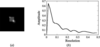Figure 2 (a) Test pattern with 64 × 64 pixels used in the computer simulations. (b) Amplitude spectrum of the test pattern showing oscillations arising from the particular shape of the test pattern. The resolution is given in units of pixel−1.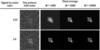Figure 3 Test patterns with 64 × 64 pixels and signal-to-noise ratios of 1/25 and 1/4 and data-set averages after 30 cycles of translational alignment for data sets containing M = 1000, 5000 and 10 000 images.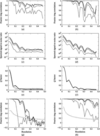Figure 4 FRC, SSNR and Q factor in resolution zones for aligned data sets of images with a signal (test pattern) and normally distributed noise. The data sets contained M = 1000 (dashed lines), 5000 (dotted lines) and 10 000 images (unbroken lines). The left column shows plots for data sets with a signal-to-noise ratio of 1/25, whereas the signal-to-noise ratio is 1/4 in the right column. Panels (g) and (h) show the FRC between the final data set averages and the original test pattern shown in Fig. 2(a), together with a plot of twice the FRC expected for pure noise (Frank, 1996). The resolution is given in units of pixel−1.

#### 4.3. The Fourier ring correlation of two independent data sets

To complete the analysis two data sets were generated each containing 5000 images of the test pattern in Fig. 2(a) and added noise with a signal-to-noise ratio of 1/4. The data sets were aligned as before, but against two separate references. In each alignment cycle, the two references were recalculated as the averages of their respective aligned data sets. An alignment with two separate references differs from the simulations in the previous section in that the images in the first data set are never aligned against the reference generated from the second data set and vice versa, thus keeping the references completely uncorrelated. The final averages were compared using the FRC (FRC1/2 in Fig. 5). The final average of the first data set was also compared to the original test pattern (FRCorig in Fig. 5; this is the same curve as in Fig. 4h for M = 5000). The FRC plots in Fig. 5show the same oscillations as seen previously. FRC1/2 is smaller than FRCorig because the former compares two noisy representations of the original test pattern whereas the latter compares a noisy representation with the noise-free test pattern. The expected variance between the two noisy averages is twice the variance between one of the noisy averages and the original test pattern. For the FRC this means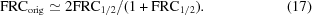A third plot (FRCestim) showing the estimated FRCorig according to (17) is also included in Fig. 5and agrees well with FRCorig except where the FRC is small (at a resolution of about 0.43) and therefore subject to increased statistical uncertainty.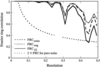Figure 5 FRC in resolution zones between averages of two data sets containing 5000 images each (FRC1/2), between the average of one data set and the original test pattern (FRCorig) and the estimated FRCorig (FRCestim), based on (17). The resolution is given in units of pixel−1.

### 5. Discussion

The aim of this study is to review common measures of resolution of structures derived by averaging images of single protein molecules or complexes. The preceding calculations and computer simulations show that depending on the resolution measure used, the indicated signal present in the final structure or in the images used to derive the structure can be fortuitous. The present study deals with the simpler case of 2D averages, but the results also apply to 3D reconstructions. The simulations in Fig. 4show that for data with a signal-to-noise ratio of 1/4 the FRC and SSNR are good indicators for the signal present in the final average. When the signal-to-noise ratio drops to 1/25 both the FRC and the SSNR still indicate a strong signal, even though comparison of the final average with the original test pattern (Fig. 4g) shows there is no signal present beyond a resolution of about 0.25. For example, for the data set containing M = 10 000 images and at a resolution of 0.3, the FRC is 0.82 (Fig. 4a) and the SSNR is 10, whereas comparison of the final average with the original test pattern in Fig. 4(g) indicates a FRC below the noise level.

Fig. 5shows that the FRC between averages of two half data sets is a true indicator of the signal present in the final average if the alignment of the two half data sets was carried out separately. However, it is common practice to combine the two half data sets to calculate a single new reference for the next alignment cycle because this doubles the signal-to-noise ratio in the reference. It is then assumed that one would still obtain a reliable resolution measurement when dividing the data into two halves (see, for example, Saxton & Baumeister, 1982). As shown in this study, this is not the case. It is therefore important to align the two half data sets against two separate references.

In the original definition of the SSNR (Unser et al., 1987), the assumption was made that the noise present in the images to be aligned is uncorrelated. This assumption is critical for the properties of the SSNR derived in the original work. If the noise in the images is uncorrelated, the effect of the fortuitously increased SSNR described here does not apply. However, it is important to note that with the alignment methods presently used in single-particle averaging, a correlation of the noise in the images cannot be avoided. Thus, using the SSNR with the current alignment methods, it is likely that the calculated signal-to-noise ratios are significantly higher than the actual signal-to-noise ratios of the averaged structure. The effect is strongest when the signal in the images is weak, a situation typically encountered in low-dose electron microscopy of frozen-hydrated specimens (Henderson, 1992). The Q factor suffers from the same problem in that it indicates a signal in the images which can be much stronger than the actual signal if the noise present in the images is correlated. Although the expectation values for the FRC, SSNR and Q factor can be estimated fairly accurately for pure noise images (Table 1), these values show a more complex behavior when a signal is present (Fig. 4). If the structure (signal) were accurately known, one could estimate the error arising from correlated noise and correct for it in a resolution plot. However, it is the very same structure one usually seeks to determine and hence a correction of the fortuitously high FRC, SSNR and Q factor is usually not possible. It follows that a non-zero correlation coefficient between a pair of noise images in a set of aligned images cannot be distinguished from a non-zero correlation coefficient arising from a real signal.

### 6. Conclusions

An important goal in modern electron microscopy of ­biological samples is the study of the 3D structure of non-crystalline samples to high resolution. Accurate measurement of the resolution of the 3D reconstruction calculated from images of single protein molecules or complexes is an essential quality assessment for the images recorded in the electron microscope, as well as for new methods to be developed to push single-particle methods to near-atomic resolution. The present study shows that commonly used measures of ­resolution, such as the Fourier ring correlation, the spectral signal-to-noise ratio or the Q factor, can yield unrealistic results. The Fourier ring correlation is a reliable indicator of a signal present in a 3D reconstruction only if the alignment of the images in the two half data sets was performed with two independent reference structures.

### Acknowledgements

The author would like to thank the W. M. Keck Foundation for financial support, and Robert Glaeser and David DeRosier for critical reading of the manuscript.

### ReferencesBIOLOGICALCRYSTALLOGRAPHY